Questions from the forum
 Q: Is it possible to render a view on a target like a model, a texture or a bmap? I'd like to use that as a mirror, for example. A: Sure, take a peek.   ENTITY* mirrorsprite;   VIEW* mirror_view;   action mirror_sprite() // attach this action to a sprite / model that will be used for the mirror {        mirrorsprite = my;                set (my, PASSABLE | DECAL); }   function mirror_startup() {        while (!mirror_sprite) {wait (1);}        mirror_view = view_create(10); // set the layer value for the new view to 10        wait (2);        set (mirror_view, SHOW);        mirror_view.size_x = 128;        mirror_view.size_y = 128; // the mirror sprite has 128x128 pixels        mirror_view.bmap = bmap_for_entity(mirrorsprite, 0);        vec_set(mirror_view.x, vector(1000, 500, 100)); // set the position of the mirror "eye"        vec_set(mirror_view.pan, vector(0, -10, 0)); // set the angles of the mirror "eye" }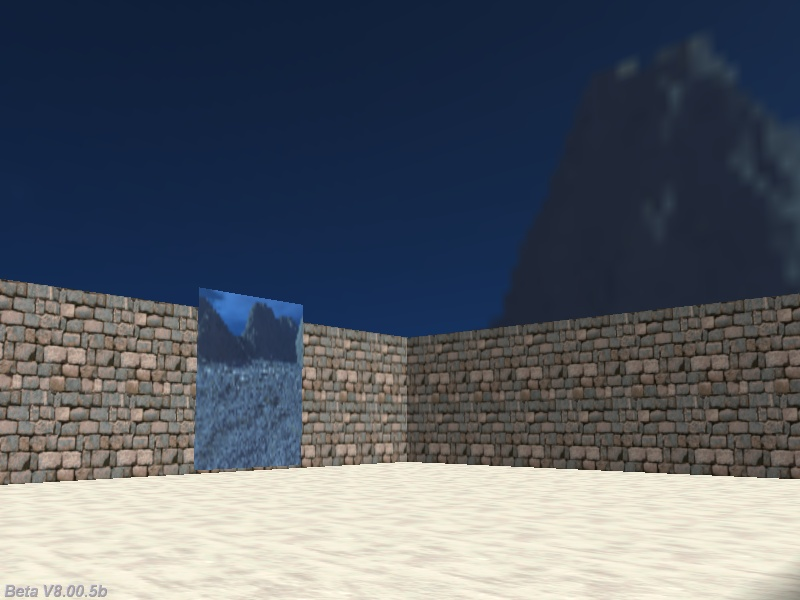Q: Is there a way to cut a view into a circle? I'd like to use that for cartoon-style cut scenes, and rectangular views are looking boring after a while... A: Use a circular model and render the view on its skin, just like in the example below.   VIEW* cutscene_view;   ENTITY* cutscene_model = {        x = 100; // tweak these values until you set the desired position and size of the circular view        y = -40;        z = 20;        type = "teler.mdl"; // use your own model name here        layer = 20;        flags2 = SHOW; // show the model }   function cut_scene_startup() {        while (!cutscene_model) {wait (1);}        cutscene_view = view_create(10); // set the layer value for the view to 10        wait (2);        set (cutscene_view, SHOW);        // the view will have 256x256 pixels; increase these values and the skin of the model if you need a higher resolution        cutscene_view.size_x = 256;        cutscene_view.size_y = 256;        cutscene_view.bmap = bmap_for_entity(cutscene_model, 0);        cutscene_view.arc = 90; // play with this value        vec_set(cutscene_view.x, vector(30, 120, -175)); // set the position of the cutscene view "eye"        vec_set(cutscene_view.pan, vector(0, 10, 0)); // set the angles of the cutscene "eye" }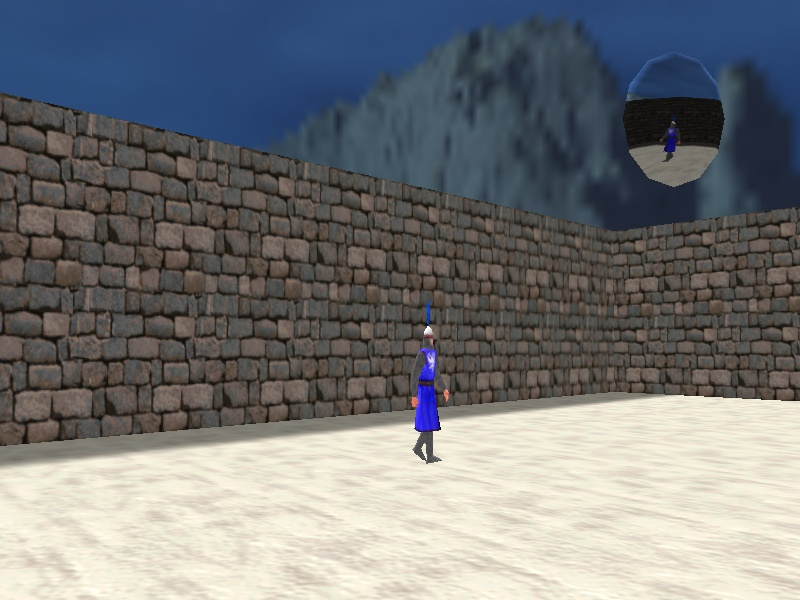Q: How can I make to so that my level blocks change their shadows dynamically using sun_light and sun_color ? A: The shadow maps for the level blocks are created when you build the level, so they can't be changed at runtime. Use camera.ambient to affect them all, or use models for your levels - they react to sun_light and sun_color.     Q: I need to display an image when my player touches an object. How do I achieve this? Any example would be helpful. A: There you go.   BMAP* pointer_tga = "pointer.tga";   PANEL* temp_pan;   function mouse_startup() {         mouse_mode = 2;        mouse_map = pointer_tga;        while (1)        {                 vec_set(mouse_pos, mouse_cursor);                wait(1);        } }   function tooltips_startup() {        var panel_on = 0;        while (1)        {                if (mouse_ent)                {                        // use different skill1 values and expand this piece of code if you want to display different images for different entities                        if (mouse_ent.skill1 == 1)                        {                                if (panel_on == 0) // the image isn't visible already? Then let's create it!                                {                                        panel_on = 1;                                        temp_pan = pan_create("bmap = circle.tga;", 10); // create a panel that uses the circle.tga bitmap and has a layer of 10                                        temp_pan.pos_x = 30; // set your desired pos_x and pos_y values for the panel                                        temp_pan.pos_y = 50;                                        temp_pan.flags |= SHOW; // and then make it visible                                }                        }                }                else // the mouse pointer didn't touch any entity?                {                        if (panel_on == 1) // the panel was created? (no need to remove it more than once)                        {                                panel_on = 0; // reset panel_on                                ptr_remove(temp_pan); // and then let's remove the panel                        }                }                wait (1);        } }     Q: Can anyone give me a tutorial about making a text box? I need to use a text box in order to ask for player's name. A: Here's an example that uses a panel as the background bitmap and a text for the input.   BMAP* pointer_tga = "pointer.tga";   STRING* name_str = "Click to input your name";   function input_name();   TEXT* name_txt = {        pos_x = 300;        pos_y = 50;        layer = 20;        string(name_str);        flags = SHOW; }   PANEL* input_pan = // used only as a background for the text box {        bmap = "hud.tga";        pos_x = 280;        pos_y = 40;        layer = 10;        on_click = input_name;        flags = SHOW; }   function input_name() {        while (mouse_left) {wait (1);} // wait until the player releases the mouse button        str_cpy(name_str, "#50"); // reset the input string        inkey(name_str); // let's input player's name }   function mouse_startup() {         mouse_mode = 2;        mouse_map = pointer_tga;        while (1)        {                 vec_set(mouse_pos, mouse_cursor);                wait(1);        } }     Q: I would like to know how to make clouds using particles. A: Here's a simple example.   BMAP* cloud_tga = "cloud.tga";   function fade_cloud(PARTICLE *p) {        p.lifespan = 100; // comment this line to have the coulds disappear after a few seconds and //        p.alpha -= 0.1 * time_step; // remove the comments for these 2 lines to have the clouds fade away as the time passes //        if (p.alpha < 0) {p.lifespan = 0;} }   function cloud_particle(PARTICLE *p) {        p->vel_x = 0.9 * (1 - random(2)); // slightly random        p->vel_y = 0.8 * (1 - random(2)); // horizontal speed        p->vel_z = 0.5 * (1 - random(2)); // and vertical speed        p.alpha = 30 + random(40); // cloud transparency, play with this value        p.bmap = cloud_tga;        p.size = 150 + random(50); // slightly random cloud size        p.flags |= (BRIGHT | MOVE);        p.event = fade_cloud; }   // Attach this action to several entities placed up high in the sky - they will become your invisible cloud generators action cloud_particles() {        set (my, INVISIBLE);        var i;        VECTOR temp;        for (i = 0; i < 100; i++) // generate 100 clouds for each entity        {                        temp.x = my.x + 500 - random(1000);                temp.y = my.y + 500 - random(1000);                                temp.z = my.z - 100 + random(200);                effect (cloud_particle, 1, temp.x, normal);        } }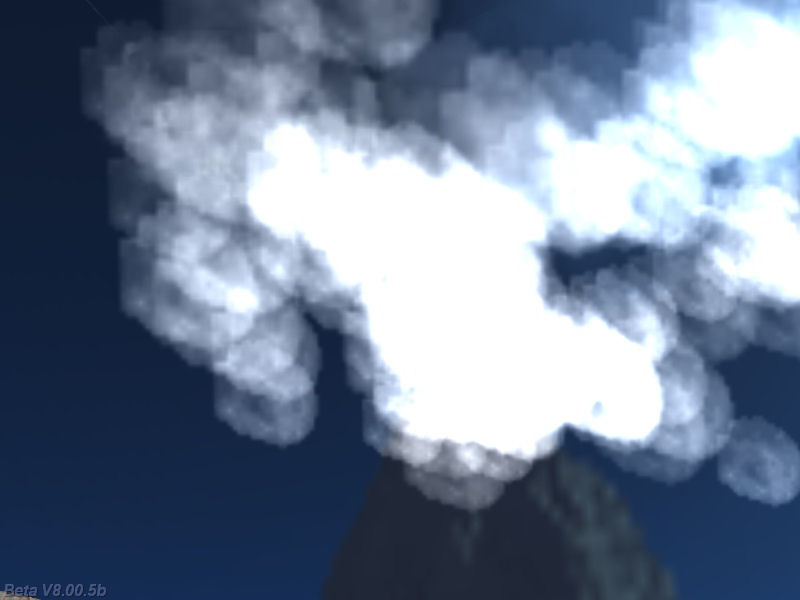Q: I'd like to create a day / night sky system that changes in real time. Can anyone help? A: Here's a simple, and yet fully functional example.   ENTITY* my_sky;   function sky_startup() {        my_sky = ent_createlayer("skycube+6.tga", SKY | CUBE | SHOW, 1);        while (1)        {                // allow the sky colors to change from 1 to 255                my_sky.red = 128 + 127 * sin(total_ticks * 0.003); // 0.003 gives the day / night transition speed                my_sky.green = my_sky.red;                my_sky.blue = my_sky.red;                wait (1);                        }         }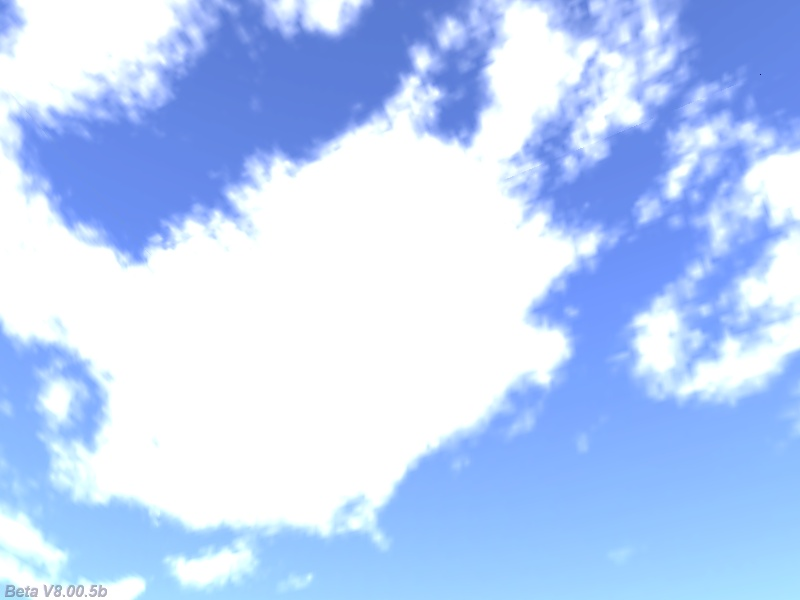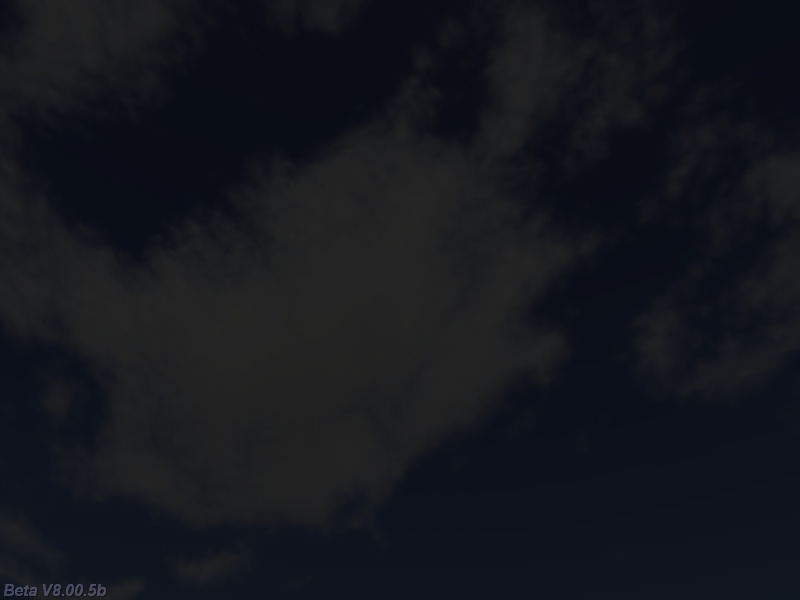Q: Is there a way to check if a certain entity was found using c_scan? There is only one "you" pointer and when I have several entities in the level, I can't tell if the entity I'm interested in was detected or not. A: There you go.   TEXT* normal_txt = {        pos_x = 100;        pos_y = 30;        string("Normal entity detected!"); }   TEXT* special_txt = {        pos_x = 300;        pos_y = 30;        string("Special entity detected!"); }   function got_scanned() {        while (event_type == EVENT_SCAN)        {                if (my.skill99 == 1) // I'm the special entity?                {                        set(special_txt, SHOW);                        }                else // detected one of the regular entities?                {                        set (normal_txt, SHOW);                }                wait (1);        }        reset (normal_txt, SHOW);        reset (special_txt, SHOW);         }   action my_regular_entities() // attach this action to your regular entities {        my.emask |= ENABLE_SCAN; // make the entity sensitive to scanning        my.event = got_scanned; }   action my_special_entity() // attach this action to your special entity {        my.skill99 = 1; // this makes your entity special (different)        my.emask |= ENABLE_SCAN; // make the entity sensitive to scanning        my.event = got_scanned; }   action players_code() // simple player / camera code, includes scanning code {        player = my; // I'm the player        set (my, INVISIBLE); // no need to see player's model in 1st person mode        while (1)        {                // move the player using the "W", "S", "A" and "D" keys; "10" = movement speed, "6" = strafing speed                c_move (my, vector(10 * (key_w - key_s) * time_step, 6 * (key_a - key_d) * time_step, 0), nullvector, GLIDE);                vec_set (camera.x, player.x); // use player's x and y for the camera as well                camera.z += 30; // place the camera 30 quants above the player on the z axis (approximate eye level)                camera.pan -= 5 * mouse_force.x * time_step; // rotate the camera around by moving the mouse                camera.tilt += 3 * mouse_force.y * time_step; // on its x and y axis                player.pan = camera.pan; // the camera and the player have the same pan angle                  // the following line makes the player scan up to 300 quants around it                c_scan(my.x, my.pan, vector(360, 180, 300), IGNORE_ME);                wait (1);        } }     Q: How can I place a panel over an entity in my game? I can't use pos_x = entity.x it because when the position is 2500 the panel is out of my screen. A: Use There you go.   PANEL* temp_pan; action panel_guy() // attach thi action to the entity that should display the panel above its head {        wait (-3);        var anim_percentage;        VECTOR temp_pos;        temp_pan = pan_create("bmap = block.pcx;", 10); // create a panel that uses the block.pcx bitmap and has a layer of 10        temp_pan.flags |= SHOW; // and then make it visible        while (1)        {                c_move (my, vector(5 * time_step, 0, 0), nullvector, GLIDE); // "5" controls the walking speed                my.pan += 4 * time_step; // this line makes the entity walk in a circle                ent_animate(my, "walk", anim_percentage, ANM_CYCLE);                anim_percentage += 4 * time_step; // "4" controls the "walk" animation speed                  vec_set (temp_pos, my.x); // copy the xyz coordinates of the entity to temp_pos                temp_pos.z += 60; // play with this value, sets the distance between the entity's origin and the panel                vec_to_screen (temp_pos, camera); // convert the 3D position to 2D screen coordinates                temp_pan.pos_x = temp_pos.x; // and then set the position of the text                temp_pan.pos_y = temp_pos.y; // on x and y                wait (1);        } }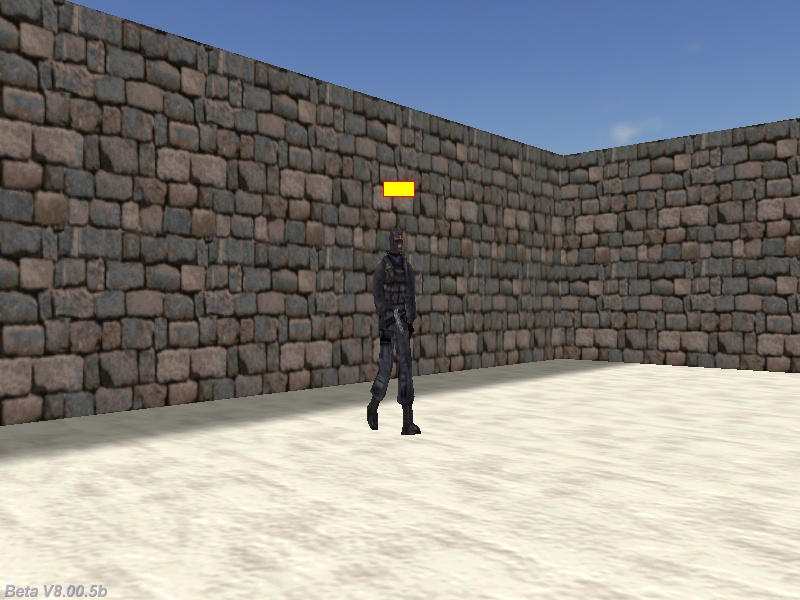Q: I have several panels with individual pointers and I need to change their position uniformly. Is there any way that I can write one function to group them together so that I don't have to write a separate pos_x / pos_y for each panel? A: Here's a snippet that will go through all the panels (you don't even need to assign pointers to them), changing their pos_x and pos_y values according to your needs.   PANEL* next_pan;   PANEL* panel_test = {        layer = 15;        pos_x = 300;        pos_y = 200;        bmap = "test.tga";        flags = SHOW; }   PANEL* panel_test2 = {        layer = 15;        pos_x = 400;        pos_y = 250;        bmap = "test.tga";        flags = SHOW; }   PANEL* panel_test3 = // feel free to add as many panels as you want {        layer = 15;        pos_x = 500;        pos_y = 300;        bmap = "test.tga";        flags = SHOW; }   // go through all the panels function move_panels(offset_x, offset_y) {        next_pan = ptr_first(panel_test);        while (next_pan)        {                 next_pan.pos_x += offset_x;                next_pan.pos_y += offset_y;                next_pan = next_pan.link.next;        } }   function move_startup() {        while (1)        {                if (key_1)                {                        while (key_1) {wait (1);}                        move_panels(20, 40); // add 20 pixels on the x axis and 40 pixels on the y axis to all the panels                }                wait (1);                        } }